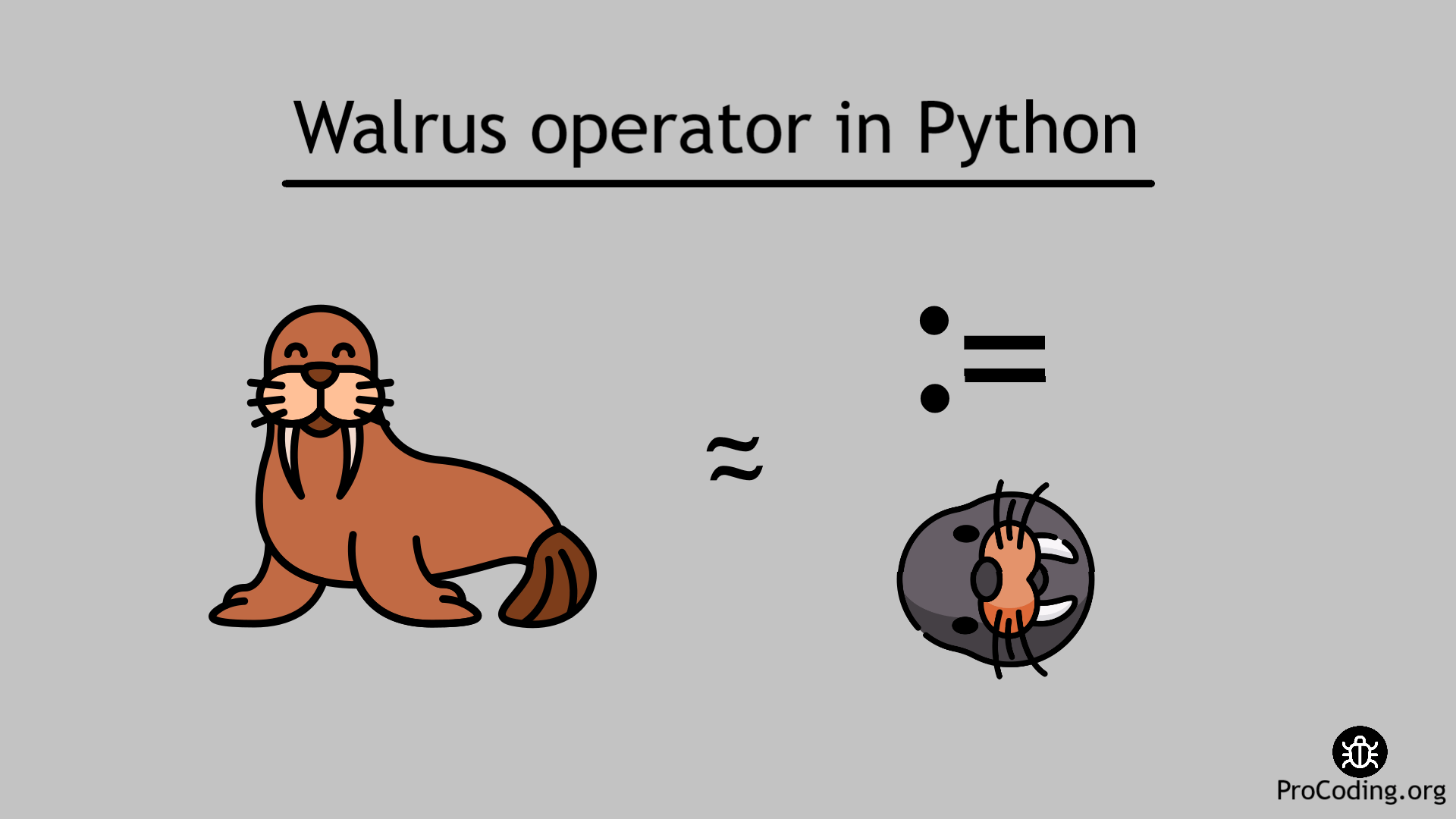# Walrus operator in Python

Category: PythonTags: python operator

Walrus operator (:=) is used as an assignment operator. It assigns value to a variable and also returns the value.
It is a new feature added in Python 3.8 version.Walrus operator (:=) is used as an assignment operator. It assigns value to a variable and also returns the value.

###### Syntax
``var_name := expression``

This operator was released as a part of PEP 572.

### How to use the Walrus operator?

To understand the use of the walrus operator consider the following code

``````my_list = [1, 2, 3, 4, 5]
n = len(my_list)
if n > 3:
print('List contains more than 3 element')
print('Length of my list is', n)``````

Output

```List contains more than 3 element
Length of my list is 5```

To reduce the number of lines in the above code we can remove the variable `n`

``````my_list = [1, 2, 3, 4, 5]
if len(my_list) > 3:
print('List contains more than 3 element')
print('Length of my list is', len(my_list))``````

Here, we have reduced the number of lines but introduced a new issue i.e., we are unnecessarily calculating the length of the list twice at line number 2 and line number 4.

To overcome this issue we can write an optimized code using walrus operator by using less number of lines and without performing any calculation multiple times.

``````my_list = [1, 2, 3, 4, 5]
if (n := len(my_list)) > 3:
print('List contains more than 3 element')
print('Length of my list is', n)``````

Output

```List contains more than 3 element
Length of my list is 5```

The walrus operator assigns value to a variable and also returns the value so that it can be used on the same line and further can be used by the variable name.

Let's take some more example to understand walrus operator properly.

##### Example

Take some inputs from the user and try to apply walrus operator here.

``````animals = []
animal = input('Enter name of an animal: ')
while animal != 'finish':
animals.append(animal)
animal = input('Enter name of an animal: ')
print(animals)``````

Output

```Enter name of an animal: Elephant
Enter name of an animal: Cow
Enter name of an animal: Dog
Enter name of an animal: Monkey
Enter name of an animal: finish
['Elephant', 'Cow', 'Dog', 'Monkey']```

Now write the same code using walrus operator

``````animals = []
while (animal := input('Enter name of an animal: ')) != 'finish':
animals.append(animal)
print(animals)``````

Output

```Enter name of an animal: Elephant
Enter name of an animal: Cow
Enter name of an animal: Dog
Enter name of an animal: Monkey
Enter name of an animal: finish
['Elephant', 'Cow', 'Dog', 'Monkey']```